# ICSE solutions for Class 10 Physics chapter 4 - Refraction through Lenses and Optical Instruments [Latest edition]

#### Chapters## Chapter 4: Refraction through Lenses and Optical Instruments

### ICSE solutions for Class 10 Physics Chapter 4 Refraction through Lenses and Optical Instruments Short Answers

What is lens?

Define the power of a lens.

What is the difference between a double convex and a bi-convex lens?

Point out the difference between a convex lens and a concave lens.

How will you decide whether a given piece of glass is a concave lens, convex lens, or a plane glass plate?

Out of the two lenses one concave and the other convex state which is a convergent or a divergent type of a lens. Give a reason for your answer.

How are the distance of object (u), the distance of image (v), and focal length (f) of a lens-related?

What will be the behaviour of a lens, if it is placed in water instead of air?

What are conjugate foci?

Name the subjective property of light related to its wavelength.

State the position of object, position of image, nature of image when: Convex lens is used as objective lens of astronomical telescope.

State the position of object, position of image, nature of image when: Convex lens is used as objective lens of photographic camera.

State the position of object, position of image, nature of image when: Convex lens is used as an erecting lens in terrestrial telescope.

What happens to the image formed by a convex lens if its lower part is blackened?

State the position of object, position of image, nature of image when: Convex lens is used in cine projector.

State the position of object, position of image, nature of image when: Convex lens is used as in searchlight.

State the position of object, position of image, nature of image when: Convex lens is used in observing biological specimens.

State the nature and position of the object on the principal axis to obtain a real and magnified image.

State the nature and position of the object on the principal axis to obtain a virtual and magnified image.

State the nature and position of the object on the principal axis to obtain a real image of the same size

We can burn a piece of paper by focussing the sun rays by using a particular type of lens. Name the type of lens used for the above purpose.

Write the three characteristics of the image formed by a convex lens of focal length 20 cm for the object at distance (i) 10 cm, (ii) 30 cm, (iii) 40 cm, (iv) 60 cm from the lens.

A lens forms an erect, magnified and virtual image of an object. name the type of lens.

A concave mirror and convex lens are held in water. What changes, if any, do you expect in their focal length?

State three characteristics of the image of an extended source, formed by a concave lens.

Do we expect any change in the position, nature, and size of the image
(i) formed by a concave lens,
(ii) with a change in the position of the object?

An object is placed in front of a lens between its optical centre and focus. The formed image is virtual, erect, and diminished. Name the lens used.

State the nature and position of the object on the principal axis to obtain. A virtual and diminished image.

Which lens can produce a real and inverted image of an object?

Ranbir claims to have obtained an image twice the size of the object with a concave lens. Is he correct? Give a reason for your answer.

In sunglasses, both of its surfaces are curved, yet their behaviour is neither like a convex lens nor like a concave lens. State the reason.

When does a ray of light falling on a lens pass through it undeviated?

A converging lens is used to obtain an image of an object placed in front of it. The inverted image is formed between F2 and 2F2 of the lens. Where is the object placed?

Define the term magnifying power of a simple microscope. How does it depend on the focal length of the lens used?

Name the lens used as a magnifying glass.

Why is the focal length of the lens, used as a magnifying glass, kept short?

Will the image formed by a magnifying glass be real or virtual?

Can a normal convex lens behave like a concave lens and vice-versa?

What information we seek by the linear magnification of the image when it is positive or negative?

Define magnification produced by a lens. State its unit.

Do we expect any change in the position, nature, and size of the image
(i) formed by a concave lens,
(ii) with a change in the position of the object?

Name the lens for which magnification can be 1. For what position of the object will the magnification be 1?

What is magnifying glass? State its two uses.

Name the lens for which magnification is always less than 1.

A convex lens produces on a screen an image twice the size of the object. If the position of image and object be interchanged, what will be the magnification then?

How is the magnification (m) produced by a lens related to the object distance (u) and the image distance (v)?

State two applications of a convex lens.

State two applications  a concave lens .

### ICSE solutions for Class 10 Physics Chapter 4 Refraction through Lenses and Optical Instruments Long Answer

Why do we say that the ‘2F’ and ‘F’ points of a convex lens can be regarded as a sort of ‘turning points’ as far as the nature of the image formed by it is concerned?

### ICSE solutions for Class 10 Physics Chapter 4 Refraction through Lenses and Optical Instruments Figure Based Short Answers

Figure Based Short Answers | Q 1

Draw a diagram to show the convergent action of a convex lens by treating it as a combination of glass block and two triangular glass prisms, with the aid of two parallel incident rays.

Figure Based Short Answers | Q 2

What is the path of the ray, after refraction, that passes through the first focus of the lens?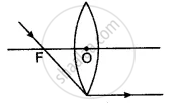Figure Based Short Answers | Q 3

What is optical centre of a thin lens? Draw diagram/diagrams to illustrate it.

Figure Based Short Answers | Q 4

Complete the ray diagram to show how the rays pass into and out of the lens?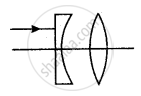Figure Based Short Answers | Q 5.1

From the nature of the refracted rays, shown in the diagram interpret the nature of the lens represented by the dotted line.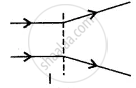Figure Based Short Answers | Q 5.2

From the nature of the refracted rays, shown in the diagram interpret the nature of the lens represented by the dotted line.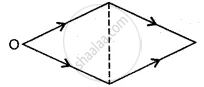Figure Based Short Answers | Q 6

Show by a diagram, the refraction of two light rays incident parallel to the principal axis on a concave lens by treating it as a combination of a glass block and two triangular glass prisms.

Figure Based Short Answers | Q 7

Where should an object be placed in front of a convex lens so as to form an inverted and enlarged image? Will the image be real or virtual? Draw a ray diagram to illustrate your answer.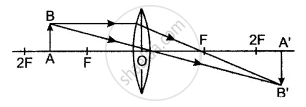Figure Based Short Answers | Q 8

Draw a ray diagram to show the formation of an inverted and diminished image of an object by a lens. Name the lens used. State the position of the object with respect to the lens used. State one more characteristic of the image.

Figure Based Short Answers | Q 9

A lens forms an upright and diminished image of an object, irrespective of its position. What kind of lens is this? Draw an outline ray diagram to show the formation of the image. State the position and one more characteristic of the image.

Figure Based Short Answers | Q 10

The following diagram shows the object O and the image I formed by a lens. Copy the diagram and on it mark the positions of the lens LL’ and focus (F). Name the lens.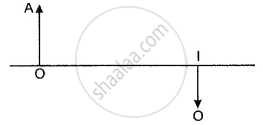Figure Based Short Answers | Q 11

In figure give below of thin concave lens, F1 and F2 are its foci, complete the path of the given ray of light after it emerges out of the lens.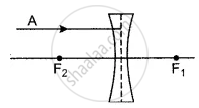Figure Based Short Answers | Q 12

Figure shows the transmitted rays through lens kept in a box. Draw the lens and complete the path of rays.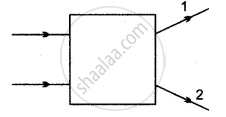Figure Based Short Answers | Q 13

Copy the following figure and complete it to show the formation of the image of the object AB. Name the lens used in the figure.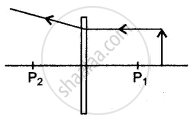Figure Based Short Answers | Q 14

In the figure given below L is a convex lens, M is a plane mirror and S is a point source of light. Rays of light from the source S return to their point of origin. Complete the ray diagram to show this. What is the point S called?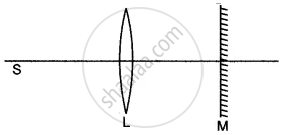Figure Based Short Answers | Q 15

An object is placed in front of a lens between its optical centre and the focus and forms a virtual, erect, and diminished image. Draw a ray diagram to show the formation of the image with the above-stated characteristics.

Figure Based Short Answers | Q 16

A candle is placed between f and 2f a convex lens. Draw a ray diagram showing the position of the image.

Figure Based Short Answers | Q 17

Draw a ray diagram to illustrate the determination of the focal length of a convex lens using an auxiliary plane mirror.

Figure Based Short Answers | Q 18

Draw the ray diagrams of a converging lens, when the object is placed at a distance greater than twice the focal length of the lens.

Figure Based Short Answers | Q 19

Draw the ray diagrams of a converging lens, when the object is placed at 2F on the principal axis. Write down the nature, position, and size of the image in both cases. Use only two rays in the diagrams.

Figure Based Short Answers | Q 20

Write down the characteristic of the image formed when the object is between F and 2F?

Figure Based Short Answers | Q 21

Write down the characteristic of the image formed when the object is between F and the lens?

Figure Based Short Answers | Q 22.1

Show with the help of a ray diagram, the path of the ray when incident normally on the first surface of the glass block, through the block and the liquid.

Figure Based Short Answers | Q 22.2

Show, using a ray diagram, how a converging lens can be used to form a virtual image.

Figure Based Short Answers | Q 23

A stick partly immersed in water appears to be bent. Draw a ray diagram to show the bending of the stick when placed in water and viewed obliquely from above.

Figure Based Short Answers | Q 24

Draw a neat diagram to explain the action of a convergent (convex) lens as a reading glass (or magnifying glass). State the characteristics of the image formed.

Figure Based Short Answers | Q 25

An object is placed in front of a convex lens such that the image formed has the same size as that of the object. Draw a ray diagram to illustrate this.

Figure Based Short Answers | Q 26

What is a simple microscope or a magnifying glass? Draw a ray diagram to show its function.

Figure Based Short Answers | Q 27

We can burn a piece of paper by focussing the sun rays by using a particular type of lens. Name the type of lens used for the above purpose. Draw a ray diagram to support your answer.

Figure Based Long Answers [Pages 1 - 2]

### ICSE solutions for Class 10 Physics Chapter 4 Refraction through Lenses and Optical Instruments Figure Based Long Answers [Pages 1 - 2]

Figure Based Long Answers | Q 1

Name the types of lenses.

Figure Based Long Answers | Q 2.1

Define the principal axis.

Figure Based Long Answers | Q 2.2

Define the optical centre of a lens.

Figure Based Long Answers | Q 3.1

Define the principal focus of a convex lens.

Figure Based Long Answers | Q 3.2

Define the principal focus of a concave lens.

Figure Based Long Answers | Q 4.1 | Page 1

Copy and complete the following ray diagram.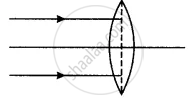Figure Based Long Answers | Q 4.1 | Page 2

Copy and complete the following ray diagram.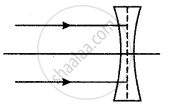Figure Based Long Answers | Q 4.2

Define the focal length.

Figure Based Long Answers | Q 5

The diagram showed a lens as a combination of one glass block and two prisms. Complete the ray diagram and show the part of the incident ray AB after passing through the lens.
(i) Name of the lens formed by the combination.
(ii) What is the line XX’ called?
(iii) Mark the focus F.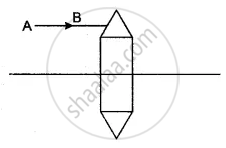Figure Based Long Answers | Q 6.1

Complete the following diagram and state what happens to the ray of light after refraction through the lens.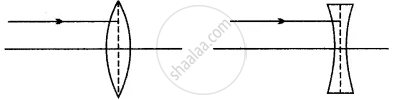Figure Based Long Answers | Q 6.2

Complete the following diagram and state what happens to the ray of light after refraction through the lens.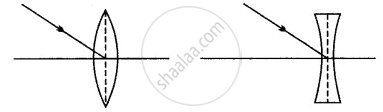Figure Based Long Answers | Q 6.3

Complete the following diagram and state what happens to the ray of light after refraction through the lens.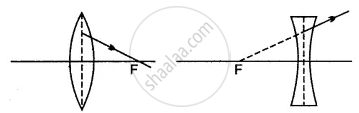Figure Based Long Answers | Q 7

The given ray diagram illustrates the experimental set up for the determination of the focal length of a converging lens using a plane mirror.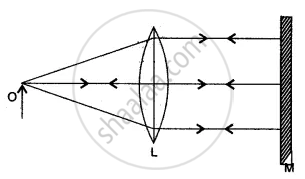(i) State the magnification of the image formed.
(ii) Write two characteristics of the image formed.
(iii) What is the name given to the distance between the object and optical centre of the lens in the above diagram?

Figure Based Long Answers | Q 8

Figure show the incident rays through the lens kept in a box. Draw the lens and complete the path of rays.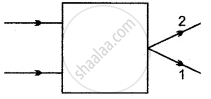Figure Based Long Answers | Q 9

Complete the diagram to show the formation of the image of the object AB.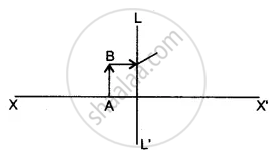(i) Name the Lens LL’.
(ii) Where is the image of the object AB formed?
(iii) State three characteristics of the image.

Figure Based Long Answers | Q 10

Draw a ray diagram to illustrate the formation of an enlarged and upright image of an object by a lens. Name the lens used. Where should the object be placed relative to the lens? Will the image be real or virtual? Name a device where this action of lens is used.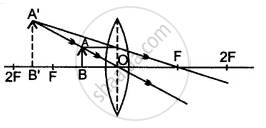Figure Based Long Answers | Q 11

An object 5 cm high is held 25 cm away from a converging lens of focal length f = 10 cm. Draw a suitable scale diagram and find the position and size of me image formed is the image real or virtual?

Figure Based Long Answers | Q 12

The diagram on right shows a ray of white light AB incident on a rectangular glass block, which is silvered at the lower surface. This ray is partly reflected and partly refracted.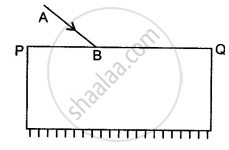(i) Copy the diagram and trace the path of the refracted and reflected ray. Show at least two rays emerging from the surface ‘PQ’
(ii) How many images are formed in the above case? Which image is the brightest?

Figure Based Long Answers | Q 13

How does a convex lens act as a ‘converging lens’?

Figure Based Long Answers | Q 14

How will you determine the focal length of a convex lens by the plane mirror method?

Figure Based Long Answers | Q 15

Define the terms principal foci and focal lengths as applied to a convex lens, and show them with the help of proper diagrams.

Figure Based Long Answers | Q 16

Diagram shows an object AB placed on the principal axis B of a convex lens placed in air. F1 and F2 are the two foci of the lens.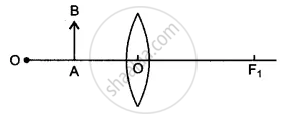(i) Copy the diagram:
Draw a ray of light starting from B and passing through O. Show the same ray after refraction by the lens. Draw another ray from B which passes through F2 after refraction by the lens. Locate the final image
(ii) Is the image real or virtual?

Figure Based Long Answers | Q 17

In the following diagram, L1 and L2 are the two convex lense placed at separation equal to the sum of focal lengths of the two lenses. A and B are the two rays of light incident on the lens L1. Complete the path of rays till they emerge out of the lens L2.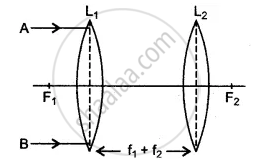What principles have you used in completing the diagram?

Figure Based Long Answers | Q 18

An erect, magnified, and a virtual image is formed when an object is placed between the optical centre and principal focus of a lens.
(i) Name the lens.
(ii) Draw a ray diagram to show the formation of the image with the above-stated characteristics.

Figure Based Long Answers | Q 19

An object AB is placed between 2F1 and F1 on the principal axis of a convex lens as shown in the diagram. Copy the diagram and using three rays starting from point A, obtain the image of the object formed by the lens.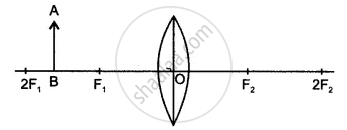Figure Based Long Answers | Q 20

How does a concave lens act as a ‘diverging lens’?

Figure Based Long Answers | Q 21

Define the term principal foci as applied to a concave lens. Illustrate your answer with the aid of proper diagrams. Show the focal length of the lens in the diagram.

Figure Based Long Answers | Q 22.1

Draw images in case of a concave lens when the object is at infinity.

Figure Based Long Answers | Q 22.2

Draw images in case of a concave lens when the object is at any point on the principal axis between the lens and infinity.

Figure Based Long Answers | Q 23

Can one bum a piece of paper in daylight by just using a convex lens instead of a match or any direct flame? Support your answer with the help of an appropriate ray diagram.

Figure Based Long Answers | Q 24

What is a magnifying glass (or a simple microscope)? Draw a ray diagram to show how a convex lens may be used as a magnifying glass. Where should the object be placed? State three characteristics of the image formed.

Figure Based Long Answers | Q 25

(i) Draw a ray diagram to show how the lens can be used as a ‘magnifying glass’? State the nature of the lens.
(ii) In what respect does the image in (i) above different from the image formed by a concave lens?

Short Numerical

### ICSE solutions for Class 10 Physics Chapter 4 Refraction through Lenses and Optical Instruments Short Numerical

Short Numerical | Q 1

A convex lens is of focal length 20 cm. Find its power.

Short Numerical | Q 2

If the lens is of focal length 25 cm. Calculate the power of the lens.

Long Numerical

### ICSE solutions for Class 10 Physics Chapter 4 Refraction through Lenses and Optical Instruments Long Numerical

Long Numerical | Q 1

If there is a convex lens of focal length 75 cm and a concave lens of focal length 40 cm, then calculate their combined power and combined focal length.

## Chapter 4: Refraction through Lenses and Optical Instruments## ICSE solutions for Class 10 Physics chapter 4 - Refraction through Lenses and Optical Instruments

ICSE solutions for Class 10 Physics chapter 4 (Refraction through Lenses and Optical Instruments) include all questions with solution and detail explanation. This will clear students doubts about any question and improve application skills while preparing for board exams. The detailed, step-by-step solutions will help you understand the concepts better and clear your confusions, if any. Shaalaa.com has the CISCE Class 10 Physics solutions in a manner that help students grasp basic concepts better and faster.

Further, we at Shaalaa.com provide such solutions so that students can prepare for written exams. ICSE textbook solutions can be a core help for self-study and acts as a perfect self-help guidance for students.

Concepts covered in Class 10 Physics chapter 4 Refraction through Lenses and Optical Instruments are Magnification Due to Spherical Lenses, Lens, Action of a Lens as a Set of Prisms, Spherical Lens, Refraction of Light Through the Equiconvex Lens and Equiconcave Lens, Guideline for Image Formation Due to Refraction Through a Convex and Concave Lens, Formation of Image by Reflection: Real and Virtual Image, Images Formed by Sperical Lenses, Concave Lens, Images Formed Due to Refraction Through a Concave Lens, Convex Lens, Images Formed Due to Refraction Through a Convex Lens, Differentiation Between Concave and Convex Lens, Sign Convention for Spherical Lenses, Lens Formula, Power of a Lens, Magnifying Glass Or Simple Microscope, Experimental Determination of Focal Length of Convex Lens.

Using ICSE Class 10 solutions Refraction through Lenses and Optical Instruments exercise by students are an easy way to prepare for the exams, as they involve solutions arranged chapter-wise also page wise. The questions involved in ICSE Solutions are important questions that can be asked in the final exam. Maximum students of CISCE Class 10 prefer ICSE Textbook Solutions to score more in exam.

Get the free view of chapter 4 Refraction through Lenses and Optical Instruments Class 10 extra questions for Class 10 Physics and can use Shaalaa.com to keep it handy for your exam preparation